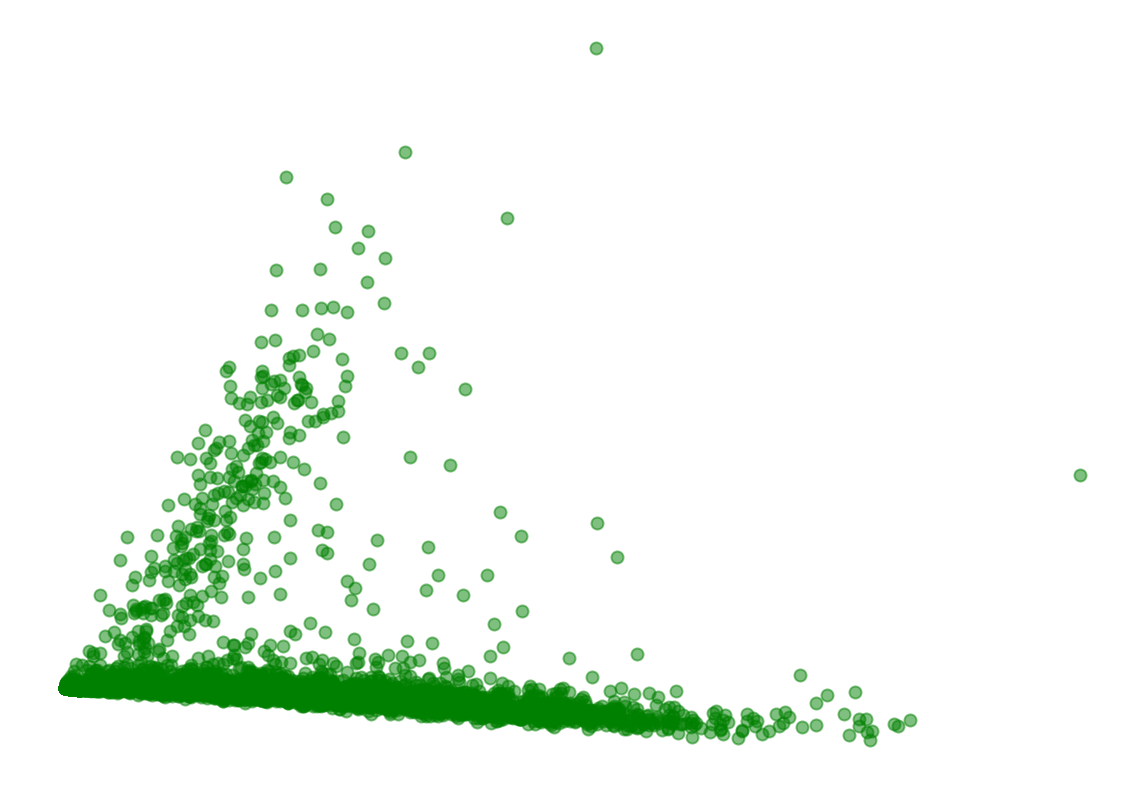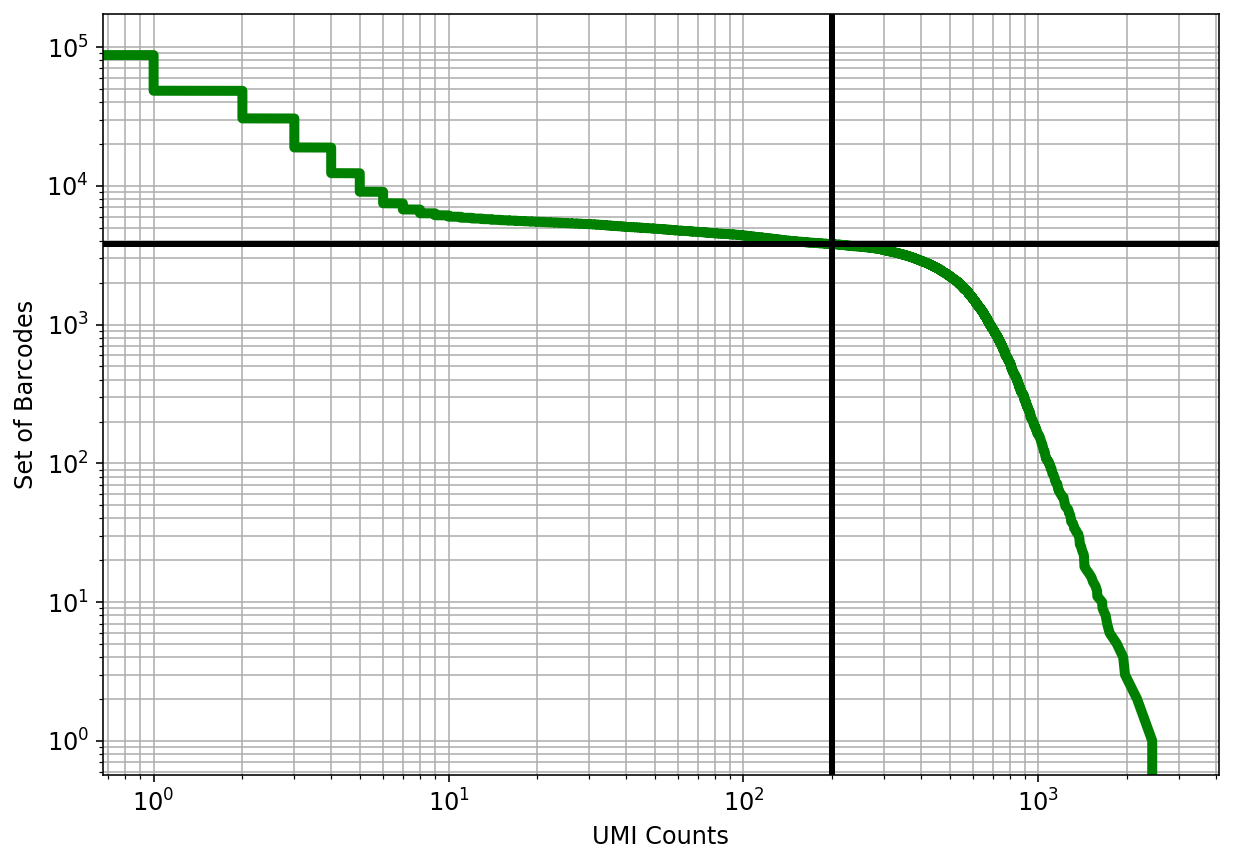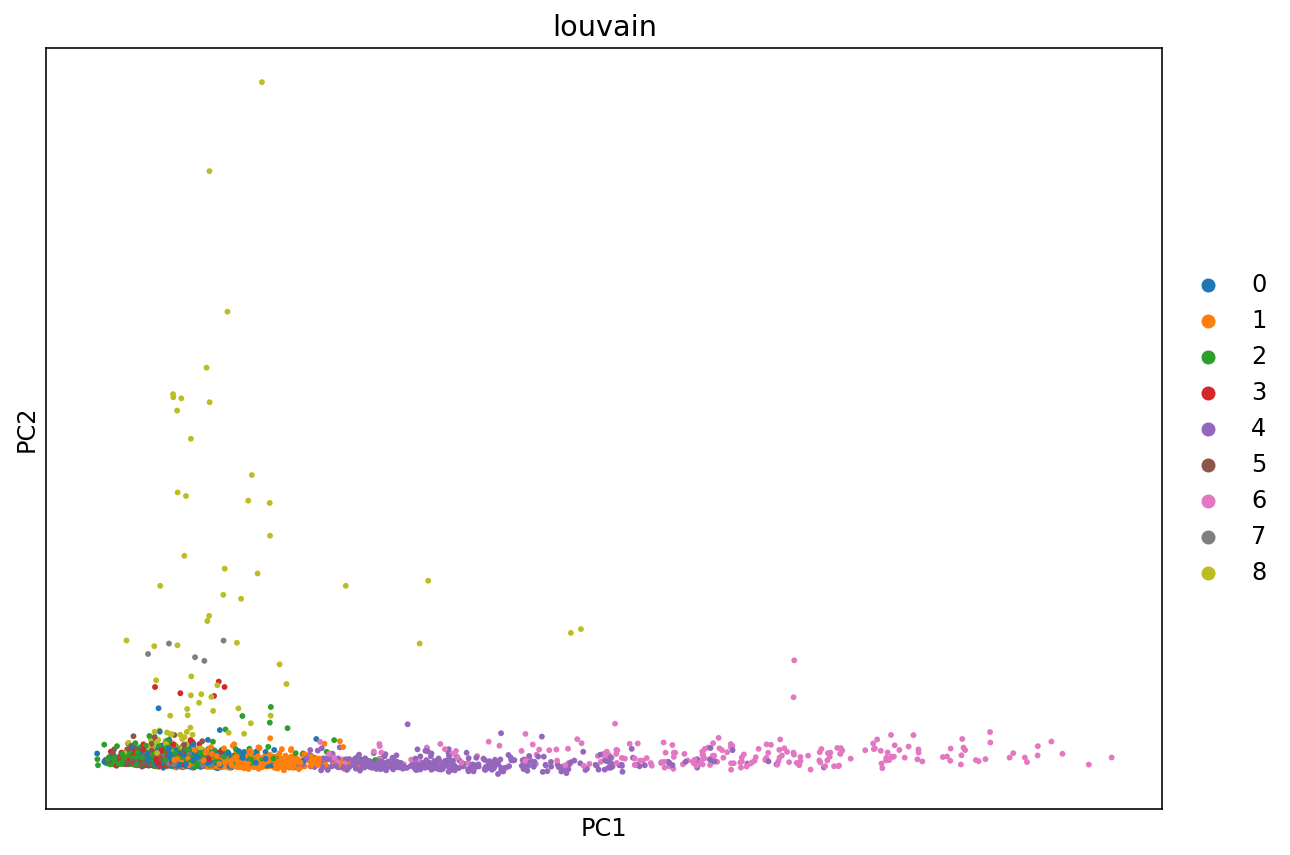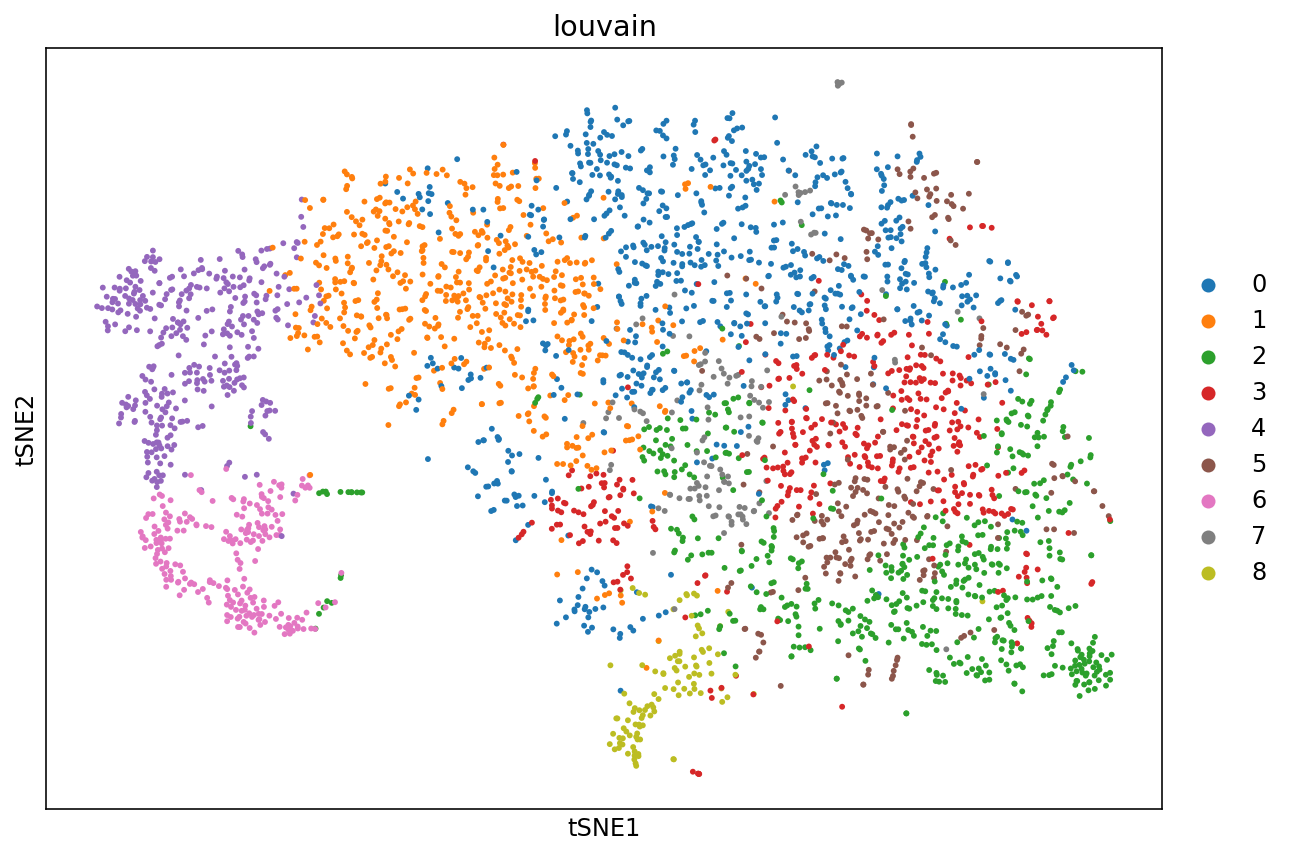# Introduction to single-cell RNA-seq II: getting started with analysis¶

This notebook demonstrates pre-processing and basic analysis of the mouse retinal cells GSE126783 dataset from Koren et al., 2019. Following pre-processing using kallisto and bustools and basic QC, the notebook demonstrates some initial analysis. The approximate running time of the notebook is about 13 minutes.

The notebook was written by Kyung Hoi (Joseph) Min, A. Sina Booeshaghi and Lior Pachter. If you use the methods in this notebook for your analysis please cite the following publications which describe the tools used in the notebook, as well as specific methods they run (these are cited inline in the notebook):

• Melsted, P., Booeshaghi, A.S. et al. Modular and efficient pre-processing of single-cell RNA-seq. bioRxiv (2019). doi:10.1101/673285
• Wolf, F. A., Angere, P. and Theis, F.J. SCANPY: large-scale single-cell gene expression data analysis. Genome Biology (2018). doi:10.1186/s13059-017-1382-0

An R notebook implementing the same analysis is available here. See the kallistobus.tools tutorials site for additional notebooks demonstrating other analyses.

## Setup¶

 ```1 2 3``` ``````# This is used to time the running of the notebook import time start_time = time.time() ``````

### Install python packages¶

 ```1 2 3 4 5``` ``````# These packages are pre-installed on Google Colab, but are included here to facilitate running this notebook locally !pip install --quiet matplotlib !pip install --quiet scikit-learn !pip install --quiet numpy !pip install --quiet scipy ``````
 ```1 2 3``` ``````%%time # `kb` is a wrapper for the kallisto and bustools program, and the kb-python package contains the kallisto and bustools executables. !pip install --quiet kb-python==0.24.1 ``````
``````[K     |████████████████████████████████| 35.4MB 82kB/s
[K     |████████████████████████████████| 133kB 39.6MB/s
[K     |████████████████████████████████| 51kB 8.3MB/s
[K     |████████████████████████████████| 112kB 45.3MB/s
[?25h  Building wheel for loompy (setup.py) ... [?25l[?25hdone
Building wheel for numpy-groupies (setup.py) ... [?25l[?25hdone
CPU times: user 107 ms, sys: 25.2 ms, total: 133 ms
Wall time: 10.2 s
``````
 ```1 2 3``` ``````%%time # Install scanpy and other packages needed for single-cell RNA-seq analysis !pip install --quiet scanpy python-igraph louvain MulticoreTSNE pybiomart ``````
``````[K     |████████████████████████████████| 2.0MB 8.2MB/s
[K     |████████████████████████████████| 3.2MB 50.3MB/s
[K     |████████████████████████████████| 2.2MB 44.0MB/s
[K     |████████████████████████████████| 81kB 11.1MB/s
[K     |████████████████████████████████| 1.1MB 48.9MB/s
[K     |████████████████████████████████| 71kB 10.0MB/s
[?25h  Building wheel for MulticoreTSNE (setup.py) ... [?25l[?25hdone
Building wheel for umap-learn (setup.py) ... [?25l[?25hdone
Building wheel for sinfo (setup.py) ... [?25l[?25hdone
Building wheel for pynndescent (setup.py) ... [?25l[?25hdone
CPU times: user 108 ms, sys: 23.3 ms, total: 132 ms
Wall time: 14.8 s
``````
 ``` 1 2 3 4 5 6 7 8 9 10 11 12``` ``````# Import packages import anndata import matplotlib import matplotlib.pyplot as plt import numpy as np import pandas as pd import scanpy as sc from sklearn.decomposition import TruncatedSVD from scipy import sparse, io matplotlib.rcParams.update({'font.size': 12}) %config InlineBackend.figure_format = 'retina' ``````

Note: We use the `-O` option for `wget` to rename the files so they can be easily identified. The notebook requires reads in fastq format; the files can be processed in gzip compressed format.

 ```1 2 3 4``` ``````%%time !wget -q https://caltech.box.com/shared/static/9u2qk1uqu6py03phooe1ti0kjd9v87pu.txt -O checksums.txt !wget -q https://caltech.box.com/shared/static/w9ww8et5o029s2e3usjzpbq8lpot29rh.gz -O SRR8599150_S1_L001_R1_001.fastq.gz !wget -q https://caltech.box.com/shared/static/ql00zyvqnpy7bf8ogdoe9zfy907guzy9.gz -O SRR8599150_S1_L001_R2_001.fastq.gz ``````
``````CPU times: user 229 ms, sys: 67.9 ms, total: 297 ms
Wall time: 52.9 s
``````
 `1` ``````# This is formatted as code ``````

 `1` ``````!md5sum -c checksums.txt --ignore-missing ``````
``````SRR8599150_S1_L001_R1_001.fastq.gz: OK
SRR8599150_S1_L001_R2_001.fastq.gz: OK
``````

Note: See this notebook for a tutorial on how to build custom transcriptome indices or indices for RNA velocity.

 ```1 2 3``` ``````%%time # This downloads a pre-built index for kallisto to use when pseudoaligning the reads !kb ref -d mouse -i index.idx -g t2g.txt ``````
``````[2021-07-07 18:15:23,071]    INFO Downloading files for mouse from https://caltech.box.com/shared/static/vcaz6cujop0xuapdmz0pplp3aoqc41si.gz to tmp/vcaz6cujop0xuapdmz0pplp3aoqc41si.gz
[2021-07-07 18:16:52,467]    INFO Extracting files from tmp/vcaz6cujop0xuapdmz0pplp3aoqc41si.gz
CPU times: user 584 ms, sys: 114 ms, total: 698 ms
Wall time: 2min 12s
``````

## Pseudoalignment and counting¶

### Run kallisto and bustools¶

The following command will generate an RNA count matrix of cells (rows) by genes (columns) in H5AD format, which is a binary format used to store Anndata objects. Notice that this requires providing the index and transcript-to-gene mapping downloaded in the previous step to the `-i` and `-g` arguments respectively. Also, since the reads were generated with the 10x Genomics Chromium Single Cell v2 Chemistry, the `-x 10xv2` argument is used. To view other supported technologies, run `kb --list`.

Note: To output a Loom file instead, replace the `--h5ad` flag with `--loom`. To obtain the raw matrix output by `kb` instead of the H5AD or Loom converted files, omit these flags.

 ```1 2 3 4``` ``````%%time # This step runs `kb` to pseudoalign the reads, and then generate the cells x gene matrix in h5ad format. !kb count -i index.idx -g t2g.txt -x 10xv2 --h5ad -t 2 \ SRR8599150_S1_L001_R1_001.fastq.gz SRR8599150_S1_L001_R2_001.fastq.gz ``````
``````[2021-07-07 18:17:35,074]    INFO Generating BUS file from
[2021-07-07 18:17:35,074]    INFO         SRR8599150_S1_L001_R1_001.fastq.gz
[2021-07-07 18:17:35,074]    INFO         SRR8599150_S1_L001_R2_001.fastq.gz
[2021-07-07 18:19:52,582]    INFO Sorting BUS file ./output.bus to tmp/output.s.bus
[2021-07-07 18:19:55,911]    INFO Whitelist not provided
[2021-07-07 18:19:55,912]    INFO Copying pre-packaged 10XV2 whitelist to .
[2021-07-07 18:19:56,015]    INFO Inspecting BUS file tmp/output.s.bus
[2021-07-07 18:19:57,425]    INFO Correcting BUS records in tmp/output.s.bus to tmp/output.s.c.bus with whitelist ./10xv2_whitelist.txt
[2021-07-07 18:20:11,880]    INFO Sorting BUS file tmp/output.s.c.bus to ./output.unfiltered.bus
[2021-07-07 18:20:14,882]    INFO Generating count matrix ./counts_unfiltered/cells_x_genes from BUS file ./output.unfiltered.bus
CPU times: user 1.05 s, sys: 159 ms, total: 1.2 s
Wall time: 2min 45s
``````

### Exercise¶

• Loom is a alternative format for storing single-cell count matrices. Output a Loom file with kb by replacing the `--h5ad` flag with `--loom`, or obtain the raw matrix output by omitting the flags
 ```1 2 3 4``` ``````%%time # # This runs `kb` to pseudoalign the reads, and then generate the cells x gene matrix in Loom format. # !kb count -i index.idx -g t2g.txt -x 10xv2 --h5ad -t 2 \ # SRR8599150_S1_L001_R1_001.fastq.gz SRR8599150_S1_L001_R2_001.fastq.gz ``````
``````CPU times: user 2 µs, sys: 0 ns, total: 2 µs
Wall time: 3.58 µs
``````

## Basic QC¶

First, the cells x genes matrix is imported into an H5AD-formatted Anndata matrix. Anndata is a convenient format for storing single-cell count matrices in Python.

 ```1 2 3``` ``````# import data adata = anndata.read('counts_unfiltered/adata.h5ad') adata ``````
``````AnnData object with n_obs × n_vars = 96775 × 55421
``````

Represent the cells in 2D with PCA

This is one type of embedding in which cells in higher dimensional gene expression space are represented in two dimensions.

 ``` 1 2 3 4 5 6 7 8 9 10 11 12``` ``````# Perform SVD tsvd = TruncatedSVD(n_components=2) tsvd.fit(adata.X) X = tsvd.transform(adata.X) # Plot the cells in the 2D PCA projection fig, ax = plt.subplots(figsize=(10, 7)) ax.scatter(X[:,0], X[:,1], alpha=0.5, c="green") plt.axis('off') plt.show() ``````### Test for library saturation¶

For each cell we ask how many genes did we detect (or see non-zero expression). The idea is that if we have "saturated" our sequencing library then increasing the number of UMI counts (x-axis) will not yield an appreciable increase in the number of genes detected (y-axis).

 ``` 1 2 3 4 5 6 7 8 9 10 11 12 13 14 15 16 17``` ``````# Create a plot showing genes detected as a function of UMI counts. fig, ax = plt.subplots(figsize=(10, 7)) x = np.asarray(adata.X.sum(axis=1))[:,0] y = np.asarray(np.sum(adata.X>0, axis=1))[:,0] ax.scatter(x, y, color="green", alpha=0.25) ax.set_xlabel("UMI Counts") ax.set_ylabel("Genes Detected") ax.set_xscale('log') ax.set_yscale('log', nonposy='clip') ax.set_xlim((0.5, 4500)) ax.set_ylim((0.5,2000)) plt.show() ``````### Examine the knee plot¶

The "knee plot" was introduced in the Drop-seq paper: - Macosko et al., Highly parallel genome-wide expression profiling of individual cells using nanoliter droplets, 2015. DOI:10.1016/j.cell.2015.05.002

In this plot cells are ordered by the number of UMI counts associated to them (shown on the x-axis), and the fraction of droplets with at least that number of cells is shown on the y-axis. The idea is that "real" cells have a certain number of UMI counts and that a threshold on the UMI counts filters those cells.

 ``` 1 2 3 4 5 6 7 8 9 10 11 12 13 14 15 16 17 18 19 20``` ``````#@title Threshold cells according to knee plot { run: "auto", vertical-output: true } cutoff = 200#@param {type:"integer"} knee = np.sort((np.array(adata.X.sum(axis=1))).flatten())[::-1] cell_set = np.arange(len(knee)) num_cells = cell_set[knee > cutoff][::-1] fig, ax = plt.subplots(figsize=(10, 7)) ax.loglog(knee, cell_set, linewidth=5, color="g") ax.axvline(x=cutoff, linewidth=3, color="k") ax.axhline(y=num_cells, linewidth=3, color="k") ax.set_xlabel("UMI Counts") ax.set_ylabel("Set of Barcodes") plt.grid(True, which="both") plt.show() ```````1` ``````print(f"{num_cells:,.0f} cells passed the {cutoff} UMI threshold") ``````
``````3,791 cells passed the 200 UMI threshold
``````

The knee plot can be used to threshold cells based on the number of UMI counts they contain. The threshold is applied at the "knee", where there is a sharp dropoff in the number of UMIs per cell. In this example we use the nunber 3979 based on the publication describing the data.

### Filter empty droplets¶

 `1` ``````adata ``````
``````AnnData object with n_obs × n_vars = 96775 × 55421
``````
 ```1 2 3``` ``````# Filter the cells according to the threshold determined from the knee plot sc.pp.filter_cells(adata, min_genes=200) sc.pp.filter_cells(adata, min_counts=knee[expected_num_cells]) ``````
 `1` ``````adata ``````
``````AnnData object with n_obs × n_vars = 3536 × 55421
obs: 'n_genes', 'n_counts'
``````

### Filtering out by mitochondrial content¶

 `1` ``````mito_ensembl_ids = sc.queries.mitochondrial_genes("mmusculus", attrname="ensembl_gene_id") ``````
 ```1 2 3 4 5 6 7``` ``````mito_genes = mito_ensembl_ids["ensembl_gene_id"].values # for each cell compute fraction of counts in mito genes vs. all genes # the `.A1` is only necessary as X is sparse (to transform to a dense array after summing) adata.obs['percent_mito'] = np.sum( adata[:, mito_genes].X, axis=1).A1 / np.sum(adata.X, axis=1).A1 # add the total counts per cell as observations-annotation to adata adata.obs['n_counts'] = adata.X.sum(axis=1).A1 ``````
 `1` ``````sc.pl.scatter(adata, x='n_counts', y='percent_mito') ```````1` ``````adata = adata[adata.obs.percent_mito < 0.03] ``````

### Filter out genes that are not present in any cells¶

 `1` ``````sc.pp.filter_genes(adata, min_cells=3) ``````
``````Trying to set attribute `.var` of view, copying.
``````
 `1` ``````adata ``````
``````AnnData object with n_obs × n_vars = 3507 × 15420
obs: 'n_genes', 'n_counts', 'percent_mito'
var: 'n_cells'
``````

### Visualizing count distributions¶

Examination of the gene count and UMI count distributions is useful QC to evaluate the quality of the library and how deeply it was sequenced.

 `1` ``````sc.pl.violin(adata, ['n_genes', 'n_counts', 'percent_mito'], jitter=0.4, multi_panel=True) ``````## Analysis¶

In this part of the tutorial, the cells are clustered by Louvain community detection.

### Normalize the counts¶

 ```1 2``` ``````sc.pp.normalize_per_cell(adata, counts_per_cell_after=1e4) sc.pp.log1p(adata) ``````

### Identify highly variable genes¶

 ```1 2 3``` ``````# flavor="cell_ranger" is consistent with Seurat and flavor="suerat" is not consistent with Seurat sc.pp.highly_variable_genes(adata, min_mean=0.01, max_mean=8, min_disp=1, n_top_genes=3000, n_bins=20, flavor="seurat") sc.pl.highly_variable_genes(adata) ```````1` ``````sc.pp.scale(adata, max_value=10) ``````

### Clustering and visualization¶

There are many algorithms for clustering cells, and while they have been compared in detail in various benchmarks (see e.g., Duo et al. 2018), there is no univerally agreed upon method. Here we demonstrate clustering using Louvain clustering, which is a popular method for clustering single-cell RNA-seq data. The method was published in

• Blondel, Vincent D; Guillaume, Jean-Loup; Lambiotte, Renaud; Lefebvre, Etienne (9 October 2008). "Fast unfolding of communities in large networks". Journal of Statistical Mechanics: Theory and Experiment. 2008 (10): P10008.
 ```1 2 3 4 5``` ``````%%time # Cluster the cells using Louvain clustering sc.tl.pca(adata, svd_solver='arpack', use_highly_variable=True, n_comps=10) sc.pp.neighbors(adata, n_neighbors=30, n_pcs=10, knn=True) sc.tl.louvain(adata) ``````
``````/usr/local/lib/python3.7/dist-packages/numba/np/ufunc/parallel.py:363: NumbaWarning: The TBB threading layer requires TBB version 2019.5 or later i.e., TBB_INTERFACE_VERSION >= 11005. Found TBB_INTERFACE_VERSION = 9107. The TBB threading layer is disabled.
warnings.warn(problem)

CPU times: user 18.2 s, sys: 804 ms, total: 19 s
Wall time: 18.8 s
``````

It is useful to revisit the PCA project with points colored by cluster. Previously we computed the PCA projection directly; here we use a function in ScanPy which does the same.

### PCA¶

 ```1 2 3``` ``````# Perform PCA and plot the projection to the first two dimensions, with points colored according to the Louvain clusters. fig, ax = plt.subplots(figsize=(10, 7)) sc.pl.pca(adata, color='louvain', ax=ax) ``````The PCA representation is the result a linear map of the data from its ambient dimension, to low dimension (in the case above 2D). While such projections are useful, there are non-linear methods that can capture non-linear geometry in the data.

### tSNE¶

t-SNE is a non-linear dimensionality reduction technique described in:

• Maaten, Laurens van der, and Geoffrey Hinton. "Visualizing data using t-SNE." Journal of machine learning research 9.Nov (2008): 2579-2605.

Here it is applied to the 10-dimensional PCA projection of the cells.

 ```1 2 3 4``` ``````%%time # Visualize cells with t-SNE. The n_pcs parameter sets the number of principal components to project to prior to # performing t-SNE sc.tl.tsne(adata, n_pcs=10) ``````
``````CPU times: user 49.6 s, sys: 109 ms, total: 49.7 s
Wall time: 25.4 s
``````
 ```1 2``` ``````fig, ax = plt.subplots(figsize=(10, 7)) sc.pl.tsne(adata, color='louvain', ax=ax) ``````## UMAP¶

UMAP stands for Uniform Manifold Approximation and Projection is a non-linear dimensionality reduction techinque described in

• Leland McInnes and John Healy and James Melville, "UMAP: Uniform Manifold Approximation and Projection for Dimension Reduction" 2018 1802.03426 arXiv stat.ML
 ```1 2 3 4``` ``````%%time # Visualize cells with t-SNE. The n_pcs parameter sets the number of principal components to project to prior to # performing t-SNE sc.tl.umap(adata) ``````
``````CPU times: user 13 s, sys: 132 ms, total: 13.2 s
Wall time: 12.6 s
``````
 ```1 2``` ``````fig, ax = plt.subplots(figsize=(10, 7)) sc.pl.umap(adata, color='louvain', ax=ax) ``````### Exercises¶

• The variance explained by each principal component is a measure of how well a projection to that component represents the data. Compute the variance explained by each component.
 ```1 2``` ``````# Compute and plot the variance explained by the PC subspaces. # sc.pl.pca_variance_ratio(adata) ``````
• In the notebook we visualized the data using t-SNE and UMAP. These two techniques can produce different two dimensional embeddings based with different parameters and even different random seeds. Change around these parameters to see how they affect the embedding.

 ``` 1 2 3 4 5 6 7 8 9 10 11 12 13 14``` ``````# %%time # # Visualize cells with UMAP. # sc.tl.tsne(adata, # n_pcs=None, # use_rep=None, # perplexity=30, # early_exaggeration=12, # learning_rate=1000, # random_state=0, # use_fast_tsne=True, # n_jobs=None, # copy=False) # fig, ax = plt.subplots(figsize=(10, 7)) # sc.pl.tsne(adata, color='louvain', ax=ax) ``````
 ``` 1 2 3 4 5 6 7 8 9 10 11 12 13 14 15 16 17 18``` ``````# %%time # # Visualize cells with UMAP. # sc.tl.umap(adata, # min_dist=0.5, # spread=1.0, # n_components=2, # maxiter=None, # alpha=1.0, # gamma=1.0, # negative_sample_rate=5, # init_pos='spectral', # random_state=0, # a=None, # b=None, # copy=False, # method='umap') # fig, ax = plt.subplots(figsize=(10, 7)) # sc.pl.umap(adata, color='louvain', ax=ax) ``````
 ```1 2``` ``````# Running time of the notebook print("{:.2f} minutes".format((time.time()-start_time)/60)) ``````
``````7.70 minutes